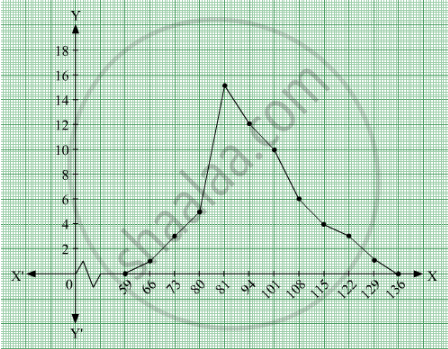# The Following Table Gives the Distribution of Iq'S (Intelligence Quotients) of 60 Pupils of Class V in a School: Draw a Frequency Polygon for the Above Data. - Mathematics

The following table gives the distribution of IQ's (intelligence quotients) of 60 pupils of class V in a school:

 IQ's: 125.5to13.25 118.5to125.5 111.5to118.5 104.5to111.5 97.5to104.5 90.5to97.5 83.5to90.5 76.5to83.5 69.5to76.5 62.5to69.5 No.of pupils: 1 3 4 6 10 12 15 5 3 1

Draw a frequency polygon for the above data.

#### Solution 1

We first draw horizontal and vertical axes. Let us consider that the horizontal and vertical axes represent the class-limits and the frequencies of the class-intervals respectively.

The given data is a continuous grouped frequency distribution with equal class-intervals. To draw the frequency polygon of the given data without using histogram, obtain the class-limits of the class intervals. Obtain the class-limits of two class-intervals of 0 frequencies, i.e. on the horizontal axis, one adjacent to the first, on its left and one adjacent to the last, on its right. These class-intervals are known as imagined class-intervals. Then plot the frequencies against class-limits.

The following table is useful to draw the frequency polygon of the given data.

 Class - Intervals Class - Marks Frequency 55.5-62.5 59 0 62.5-69.5

We represent class marks on X-axis on a suitable scale and the frequencies on Y-axis on a suitable scale.
To obtain the frequency polygon we plot the points (66, 1), (73, 3), (80, 5), (87, 15), (94, 12), (101, 10), (108, 6), (115, 4), (122, 3), (129, 1).
Now we join the plotted points by line segments. The end points (66, 1) and (129, 1) are joined to the mid points (59, 0) and ( 136, 0) respectively of imagined class intervals to obtain the frequency polygon.#### Solution 2

We first draw horizontal and vertical axes. Let us consider that the horizontal and vertical axes represent the class-limits and the frequencies of the class-intervals respectively.

The given data is a continuous grouped frequency distribution with equal class-intervals. To draw the frequency polygon of the given data without using histogram, obtain the class-limits of the class intervals. Obtain the class-limits of two class-intervals of 0 frequencies, i.e. on the horizontal axis, one adjacent to the first, on its left and one adjacent to the last, on its right. These class-intervals are known as imagined class-intervals. Then plot the frequencies against class-limits.

The following table is useful to draw the frequency polygon of the given data.

 Class - Intervals Class - Marks Frequency 55.5-62.5 59 0 62.5-69.5 66 1 69.5 - 76.5 73 3 76.5 - 83.5 80 5 83.5 - 90.5 87 15 90.5 - 97.5 94 12 97.5-104.5 101 10 104-111.5 108 6 111.5 - 118.5 115 4 118.5-125.5 122 3 125.5-132.5 129 1 132.5-139.5 136 0

We represent class marks on X-axis on a suitable scale and the frequencies on Y-axis on a suitable scale.
To obtain the frequency polygon we plot the points (66, 1), (73, 3), (80, 5), (87, 15), (94, 12), (101, 10), (108, 6), (115, 4), (122, 3), (129, 1).
Now we join the plotted points by line segments. The end points (66, 1) and (129, 1) are joined to the mid points (59, 0) and ( 136, 0) respectively of imagined class intervals to obtain the frequency polygon.Concept: Graphical Representation of Data
Is there an error in this question or solution?

#### APPEARS IN

RD Sharma Mathematics for Class 9
Chapter 23 Graphical Representation of Statistical Data
Exercise 23.3 | Q 6 | Page 42

Share